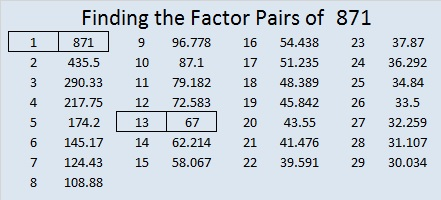# 871 Is the Smallest Number That Is the Sum of 3 Triangular Numbers 23 Different Ways

OEIS.org alerted me to the fact that 871 is the smallest number that is the sum of three triangular numbers 23 different ways. I set out to find those 23 ways. We’ll have to count 0 as the 0th triangular number for four of them, but here are those record-breaking 23 ways:871 is the hypotenuse of Pythagorean triple 335-804-871 which is (5-12-13) times 67.

871 is also repdigit 111 in BASE 29 because 1(29²) + 1(29¹) + 1(29º) = 871.

• 871 is a composite number.
• Prime factorization: 871 = 13 × 67
• The exponents in the prime factorization are 1 and 1. Adding one to each and multiplying we get (1 + 1)(1 + 1) = 2 × 2 = 4. Therefore 871 has exactly 4 factors.
• Factors of 871: 1, 13, 67, 871
• Factor pairs: 871 = 1 × 871 or 13 × 67
• 871 has no square factors that allow its square root to be simplified. √871 ≈ 29.512709This site uses Akismet to reduce spam. Learn how your comment data is processed.